The REG Procedure
 RESTRICT Statement
RESTRICT equation <, ..., equation> ;

A RESTRICT statement is used to place restrictions on the parameter estimates in the MODEL preceding it. More than one RESTRICT statement can follow each MODEL statement. Each RESTRICT statement replaces any previous RESTRICT statement. To lift all restrictions on a model, submit a new MODEL statement. If there are several restrictions, separate them with commas. The statement

```restrict equation1=equation2=equation3;
```

is equivalent to imposing the two restrictions

```restrict equation1=equation2;
restrict equation2=equation3;
```

Each restriction is written as a linear equation and can be written as

equation

or

equation = equation

The form of each equation is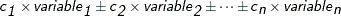where the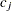’s are constants and the variable’s are any regressor variables.

When no equal sign appears, the linear combination is set equal to zero. Each variable name mentioned must be a variable in the MODEL statement to which the RESTRICT statement refers. The keyword INTERCEPT can also be used as a variable name, and it refers to the intercept parameter in the regression model.

Note that the parameters associated with the variables are restricted, not the variables themselves. Restrictions should be consistent and not redundant.

Examples of valid RESTRICT statements include the following:

```restrict x1;
restrict a+b=l;
restrict a=b=c;
restrict a=b, b=c;
restrict 2*f=g+h, intercept+f=0;
restrict f=g=h=intercept;
```

The third and fourth statements in this list produce identical restrictions. You cannot specify

```restrict f-g=0,
f-intercept=0,
g-intercept=1;
```

because the three restrictions are not consistent. If these restrictions are included in a RESTRICT statement, one of the restrict parameters is set to zero and has zero degrees of freedom, indicating that PROC REG is unable to apply a restriction.

The restrictions usually operate even if the model is not of full rank. Check to ensure that DF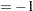for each restriction. In addition, the model DF should decrease by 1 for each restriction.

The parameter estimates are those that minimize the quadratic criterion (SSE) subject to the restrictions. If a restriction cannot be applied, its parameter value and degrees of freedom are listed as zero.

The method used for restricting the parameter estimates is to introduce a Lagrangian parameter for each restriction (Pringle and Rayner 1971). The estimates of these parameters are displayed with test statistics. Note that thestatistic reported for the Lagrangian parameters does not follow a Student’sdistribution, but its square follows a beta distribution (LaMotte 1994). The-value for these parameters is computed using the beta distribution.

The Lagrangian parameter
measures the sensitivity of the SSE to the restriction constant. If the restriction constant is changed by a small amount, the SSE is changed by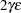. Theratio tests the significance of the restrictions. Ifis zero, the restricted estimates are the same as the unrestricted estimates, and a change in the restriction constant in either direction increases the SSE.

RESTRICT statements are ignored if the PCOMIT= or RIDGE= option is specified in the PROC REG statement.Previous Page | Next Page | Top of Page